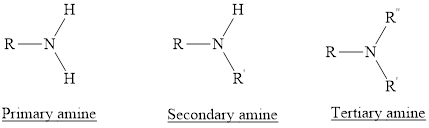Chapter 23, Problem 47PS

Chapter
Section
Textbook Problem

Write the formula, and draw the structure, for each of the following amines:(a) ethylamine(b) dipropylamine(c) butyldimethylamine(d) triethylamine

(a)

Interpretation Introduction

Interpretation: For the given amines structural formula has to be drawn and also their molecular formula has to be given.

Concept introduction:

Amines are the hydrocabons which have nitrogen (N) heteroatom. The nitrogen atom is substituted by alkyl groups which can be aliphatic, aromatic or cyclic. On the basis of number of alkyl groups three types of amines are possible.

(1) Primary Amines: The nitrogen atoms is substituted by one alkyl group and two hydrogen atoms are also attached to nitrogen atom is called as primary amine. For example,

(2) Secondary Amines: The nitrogen atoms is substituted by two alkyl groups and one hydrogen atom is also attached to nitrogen atom is called as secondary amine.

(3) Tertiary Amines: The nitrogen atoms is substituted by three alkyl groups and no hydrogen atom is attached to nitrogen atom is called as tertiary amine.Explanation

The molecular formula of the given amine can be written by using its given name ethylamine.

The ethyl group (C2H5) is substituted to the nitrogen atom and it is primary amine as no other alkyl groups are attached to nitrogen atom

(b)

Interpretation Introduction

Interpretation: For the given amines structural formula has to be drawn and also their molecular formula has to be given.

Concept introduction:

Amines are the hydrocabons which have nitrogen (N) heteroatom. The nitrogen atom is substituted by alkyl groups which can be aliphatic, aromatic or cyclic. On the basis of number of alkyl groups three types of amines are possible.

(1) Primary Amines: The nitrogen atoms is substituted by one alkyl group and two hydrogen atoms are also attached to nitrogen atom is called as primary amine. For example,

(2) Secondary Amines: The nitrogen atoms is substituted by two alkyl groups and one hydrogen atom is also attached to nitrogen atom is called as secondary amine.

(3) Tertiary Amines: The nitrogen atoms is substituted by three alkyl groups and no hydrogen atom is attached to nitrogen atom is called as tertiary amine.(c)

Interpretation Introduction

Interpretation: For the given amines structural formula has to be drawn and also their molecular formula has to be given.

Concept introduction:

Amines are the hydrocabons which have nitrogen (N) heteroatom. The nitrogen atom is substituted by alkyl groups which can be aliphatic, aromatic or cyclic. On the basis of number of alkyl groups three types of amines are possible.

(1) Primary Amines: The nitrogen atoms is substituted by one alkyl group and two hydrogen atoms are also attached to nitrogen atom is called as primary amine. For example,

(2) Secondary Amines: The nitrogen atoms is substituted by two alkyl groups and one hydrogen atom is also attached to nitrogen atom is called as secondary amine.

(3) Tertiary Amines: The nitrogen atoms is substituted by three alkyl groups and no hydrogen atom is attached to nitrogen atom is called as tertiary amine.(d)

Interpretation Introduction

Interpretation: For the given amines structural formula has to be drawn and also their molecular formula has to be given.

Concept introduction:

Amines are the hydrocabons which have nitrogen (N) heteroatom. The nitrogen atom is substituted by alkyl groups which can be aliphatic, aromatic or cyclic. On the basis of number of alkyl groups three types of amines are possible.

(1) Primary Amines: The nitrogen atoms is substituted by one alkyl group and two hydrogen atoms are also attached to nitrogen atom is called as primary amine. For example,

(2) Secondary Amines: The nitrogen atoms is substituted by two alkyl groups and one hydrogen atom is also attached to nitrogen atom is called as secondary amine.

(3) Tertiary Amines: The nitrogen atoms is substituted by three alkyl groups and no hydrogen atom is attached to nitrogen atom is called as tertiary amine.Still sussing out bartleby?

Check out a sample textbook solution.

See a sample solution

The Solution to Your Study Problems

Bartleby provides explanations to thousands of textbook problems written by our experts, many with advanced degrees!

Get Started